By 4th grade, students need to stop counting on their fingers and be able to recall math facts immediately. If not, then they will find it hard to learn multiplication and division. Rocket Math offers 4th grade math worksheets that will push your students towards success and prepare them for 5th grade.## What should a 4th grader know in math?

By the end of fourth grade, students should have memorized all four basic operations of math facts: addition, subtraction, multiplication, and division. Typically, students in fourth grade learn division. However, if your fourth grade students haven’t mastered multiplication, you must help them develop multiplication math fact automaticity first and then teach them, division, if there is enough time in the school year. Without math fact fluency in multiplication, students will struggle with division and learning all higher-order forms of math including algebra.

The only way for students to successfully memorize math facts is systematical. Practicing random math facts or playing math games will never help students memorize. They will simply count the facts out over and over again–coming to hate math.

## How how to measure math fact fluency

Not sure if your students are fluent in multiplication math facts and are ready to learn division? If you see a student counting on their fingers, it’s a sure bet they have NOT memorized these basic single-digit facts. If you don’t know for sure, here’s our free resource with all you need to pre-test students on math facts.

## Why Rocket Math Worksheets help students develop math fact mastery

Rocket Math’s Worksheet Program is very different from any other math facts practice worksheets.

The program only teaches a couple of math facts at a time, helping students succeed every day.

Once students learn the correct answers, the Worksheet Program includes a lot of fast-paced practice. With this “learn first, practice second” method, students easily commit their math facts to memory.

The program is graduated and progressive. It mixes newly memorized math facts with previously memorized math facts, helping students develop over time the ability to answer more than 400 math facts instantly from recall.

When students develop automaticity (facts so well learned that their recall is instantaneous), all math becomes easier.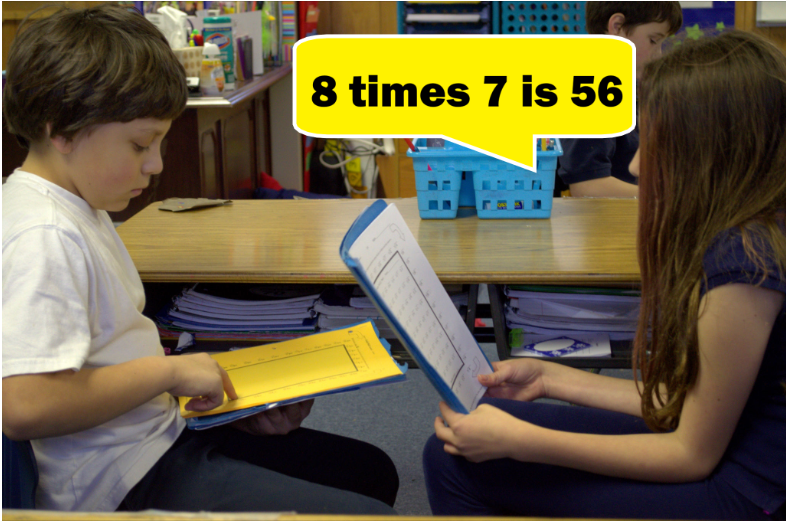A unique component of the Worksheet Program is oral student practice. This allows students to receive corrective feedback from their partner, who has the answer key. You cannot learn without corrective feedback from either a person or a computer. Having teachers give corrective feedback by checking and grading written practice is very time-consuming, as well as being too late for effective learning. Having student partners with answer keys correct immediately, during practice, means teachers only have to grade check-point tests instead of monitoring daily practice–a huge plus for time-strapped teachers!

The Worksheet Program is designed to build strong multiplication fact fluency and recall. Memorizing these facts avoids the problem of students having to look up facts in times tables, over and over again.

## How to teach MULTIPLICATION with Rocket Math’s 4th Grade Math Worksheets

Since many students begin fourth grade without mastery of multiplication facts, let’s start with Rocket Math’s first multiplication worksheet of Set A. As you can see, the worksheet has only “1 times” problems, such as 3 times 1, and 1 times 2. These are very easy, and students can typically answer them quickly, with no hesitation.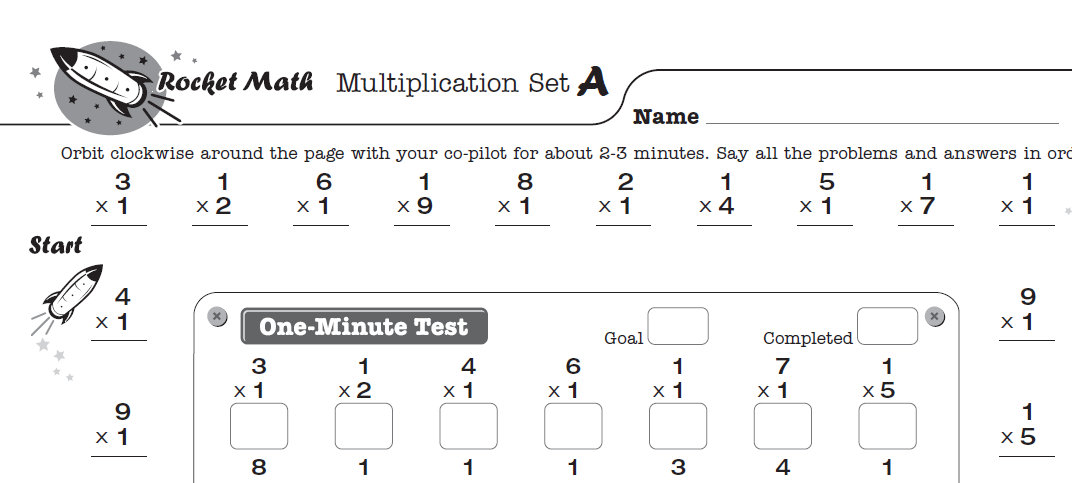First, students practice orally with a partner around the outside of the worksheet. For example, one student practices saying the problems and the answers while the other student offers corrections. You need to teach students that the checker’s role is to correct any fact that isn’t answered instantly without hesitation. The checker needs to follow this special correction procedure.

After two to three minutes of oral practice, students should switch roles and help their partner practice.

Finally, all students take a one-minute test of these facts on the inside of the worksheet. They will be able to write the answers to those facts as fast as their fingers can carry them.

## Writing Speed Test

Before beginning the Worksheet Program, students need to establish goals with a writing speed test. Students who can answer the problems in the one-minute daily test at their established speed will pass on to learn the next set of facts the following day.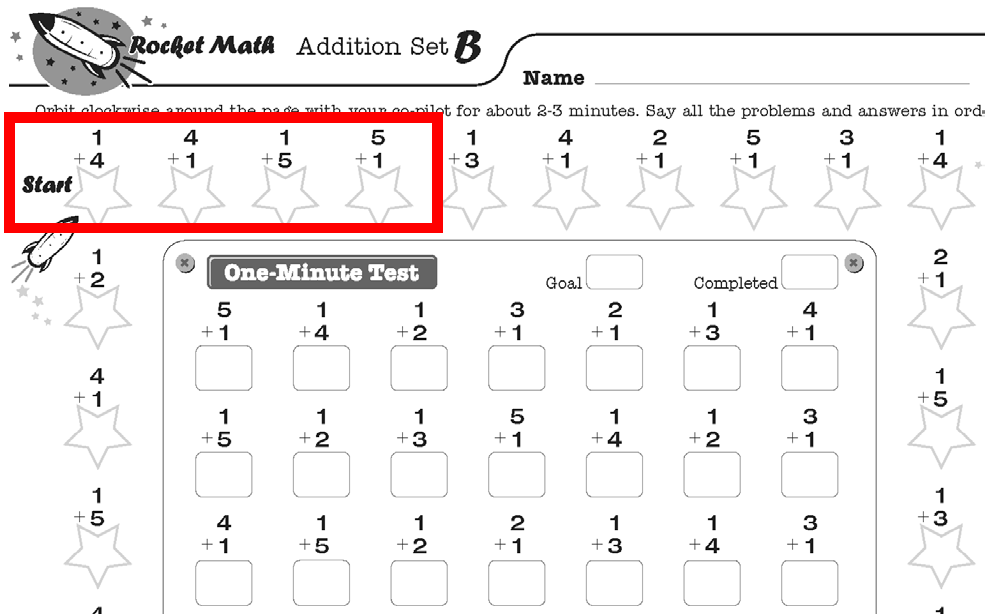Once your students master Set A, move on to Set B, then Set C, and so on. Get all of the Multiplication 1s to 9s worksheets from rocketmath.com. Each worksheet follows the same procedure:

1. Students practice saying the answers to the “outer ring” problems out loud with a partner.
2. Partners follow the special correction procedure for any fact that isn’t answered instantly without hesitation.
3. After 2-3 minutes of partner practice, all students take a one-minute test of the recently learned facts located on the inside part of the worksheet.
4. Students that answer the correct amount of math fact problems in 1 minute (based on their writing speed) progress to the next worksheet set. Students that fail the test, repeat steps 1-4 using the same worksheet set the following day.

It usually only takes students a few days to memorize a set.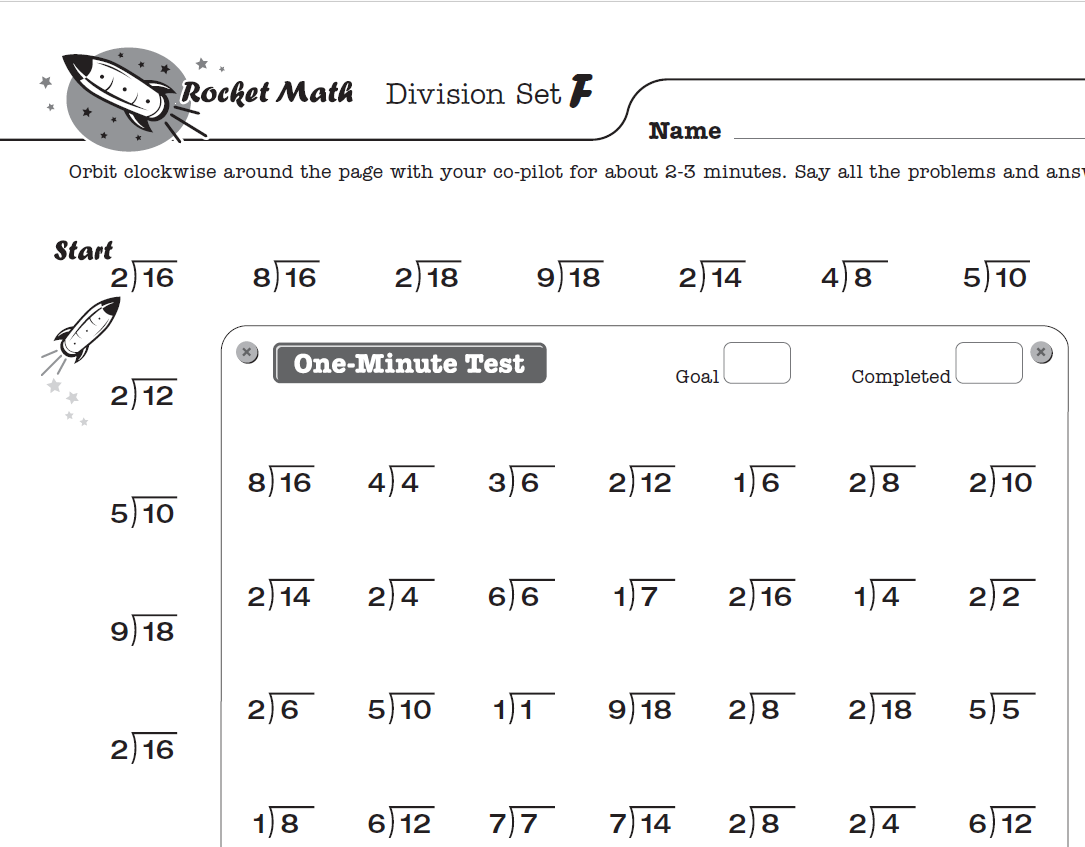## How to teach DIVISION with Rocket Math’s 4th Grade Math Worksheets

Once your fourth graders master multiplication math facts, it’s time to teach them division.

To teach your fourth graders division with Rocket Math’s worksheets, use the Division 1s through 9s worksheet and follow the same procedure described in the previous section on multiplication.

The Common Core suggested that students learn math facts up through the 12s. Rocket Math has two Worksheet Programs that do just that. One is Multiplication 10s, 11s, 12s. and the other is Division 10s, 11s, 12s. Each builds upon and reviews facts from the 1s-9s program while keeping learning fun for students who learn faster and need additional challenges.## Fact Family Worksheets are another way to study facts

Fact families worksheets provide an alternative way for fourth graders to learn multiplication and division facts. A fact family in multiplication and division is 4 x 3, 3 x 4, 12 ÷ 3, and 12 ÷ 4. By learning these four facts simultaneously, students can internalize the reciprocal nature of multiplication and division. This is also a viable way for students to learn facts initially if that is the teacher’s preference. This can also be a great review for students who begin the year having mastered multiplication and division.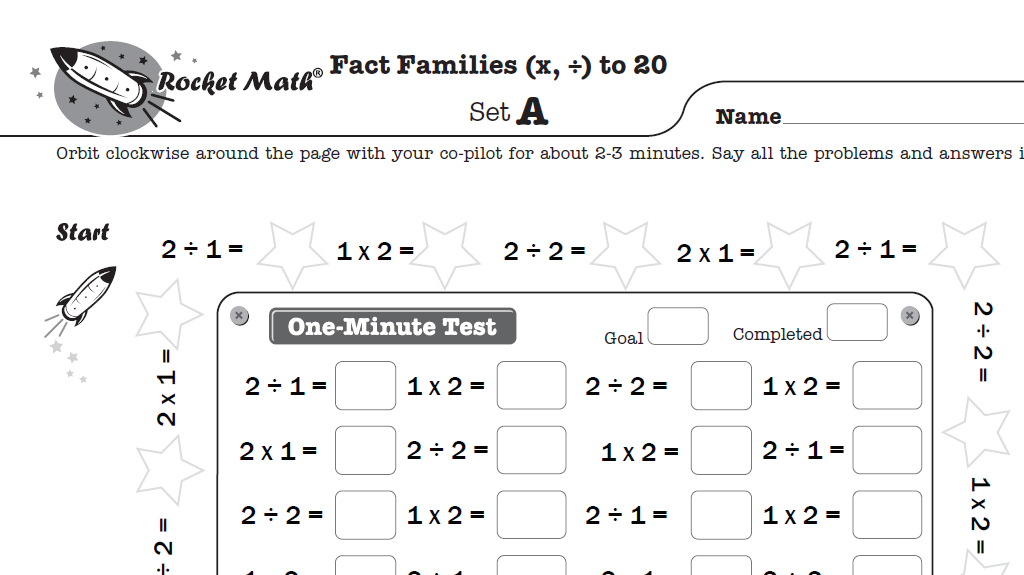### Rocket Math Worksheet Program’s specially designed Fact Families

Much like Rocket Math’s single operation worksheets, each Fact Family worksheet in the Rocket Math program asks students to memorize only one fact family at a time. You can see the first fact family students learn in Set A is 1 x 2, 2 x 1, 2 ÷ 1, and 2 ÷ 2. Each set in this program introduces only one fact family to help students easily memorize fact families and work quickly through Sets A to Z..

Rocket Math’s Worksheet Program starts with the first level of Multiplication and Division Fact Families to 20, followed by Multiplication and Division Fact Families from 21. Practicing with fact families reinforces the reciprocal nature of multiplication and division and gives extra practice to increase fluency.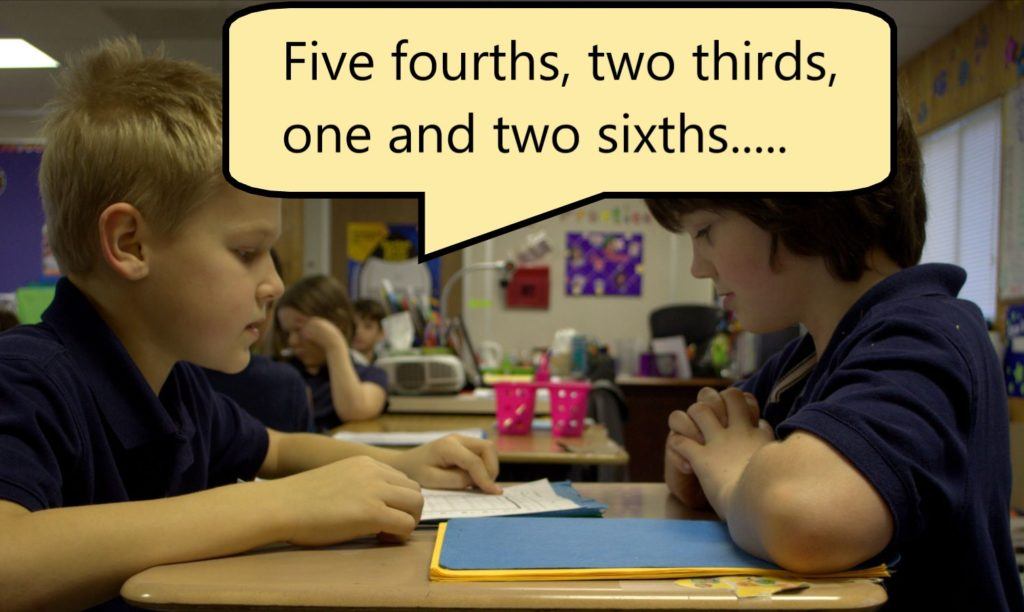## 4th grade worksheets for learning fractions and factor pairs

Rocket Math Worksheet Program works through a daily routine of oral practice with peers and one-minute tests to determine how fast a student should progress through the A to Z steps. By establishing a daily routine, students can use it to study fractions, factors, and decimals in the more advanced pre-algebra programs available in Rocket Math. These will seriously give students a “leg-up” when they come to pre-algebra concepts that students find slow or cumbersome.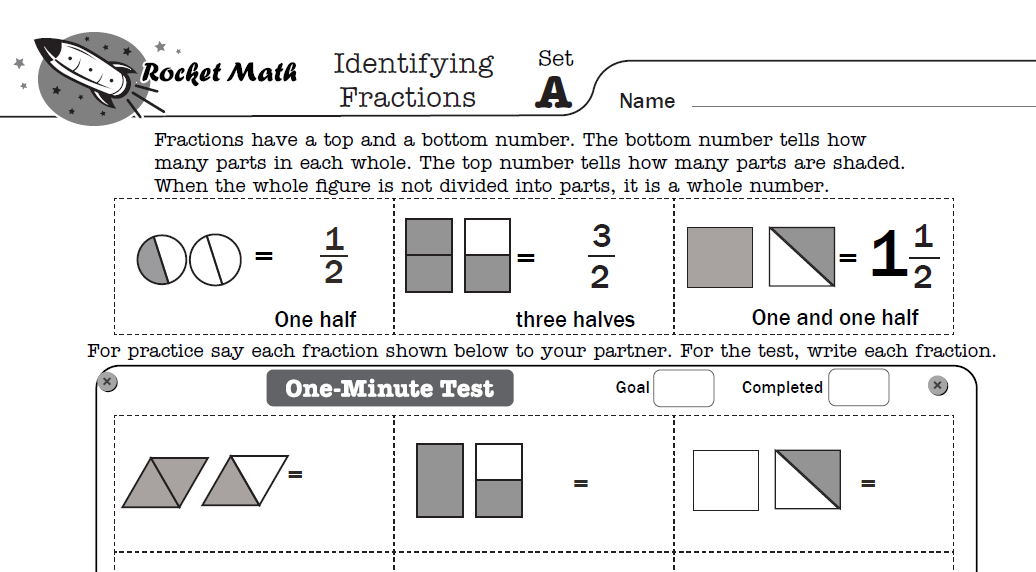### Identifying Fractions Worksheets

One such Rocket Math program is the Identifying Fractions Worksheet program. In it, students learn to identify pictures of proper fractions, improper fractions, and mixed numbers. Each A to Z set teaches a new set of three closely related fractions so that students learn to identify the fractions quickly and easily. Students learn this rule: Fractions have a top and a bottom number. The bottom number tells how many parts in each whole. The top number tells how many parts are shaded. When the whole figure is not divided into parts, it is a whole number. The program also presents whole numbers to identify mixed in with fractions. If you have ever seen students struggle with identifying fractions when they are looking at them, you’ll appreciate the power of these worksheets.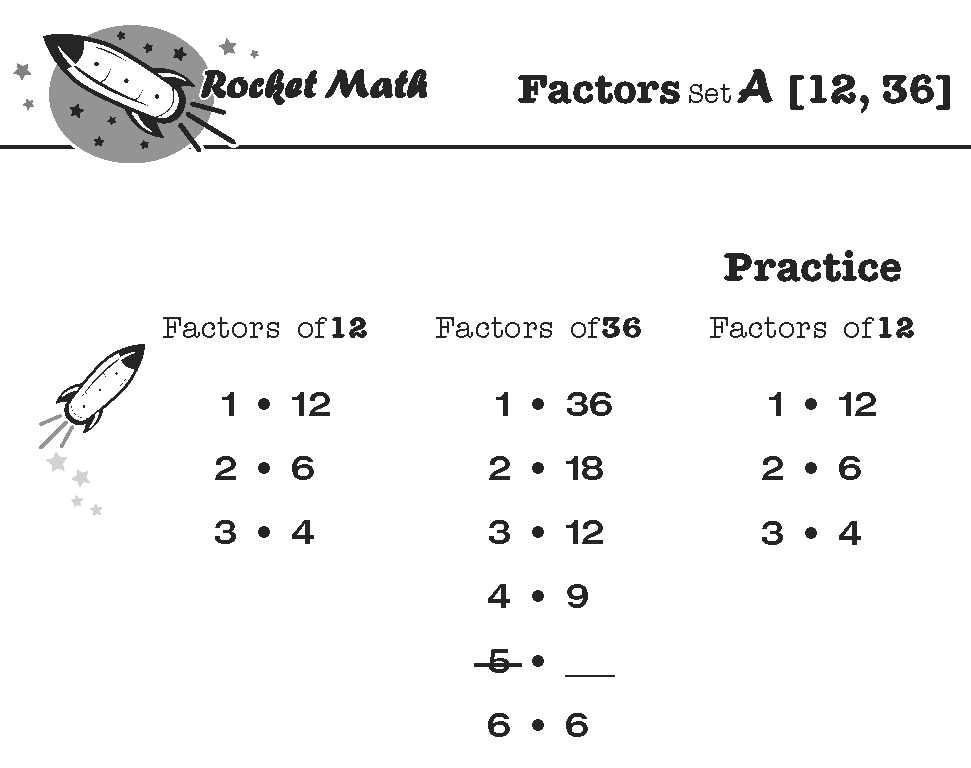### Factors (how to find all the factor pairs) Worksheets

Rocket Math Worksheet Program Factors have worksheets to quickly teach students how to name all the factor pairs of common numbers. What are the factors of 36? Answer: 1 x 36, 2 x 18, 3 x 12, 4 x 9, 6 x 6. This is what students learn by memory. Here’s a blog about the foolproof method that students learn. Students practice with a partner, take a daily one-minute timing, fill in a Rocket Chart, just like regular Rocket Math. Simplifying fractions will be a breeze after students learn this easy method and practice finding all the factors of these common numbers.

Students learn all the factors for these numbers in this sequence: 12, 36, 24, 48, 18, 32, 16, 64, 10, 40, 20, 72, 8, 25, 50, 6, 21, 30, 60, 15, 45, and 100. Click here to learn “How to Factor” from Dr. Don’s Educreations lesson on the web.

### Equivalent Fractions

Students need to know that six-eighths is equivalent to three-fourths and that four-twelfths is equivalent to one-third. While they can calculate these, it is very helpful to know the most common equivalent fractions by memory. One of the most common problems students have with fractions is not “reducing their answers to simplest form.”

Here’s a 5-minute Educreations lesson on How the Equivalent Fractions program works.

The Equivalent Fractions Worksheet Program is part of the Universal Level subscription to the Worksheet Program. Equivalent fractions will help students commit 100 common equivalent fractions to memory. Each set (A through Z) has four fractions, which are displayed on a fraction number line. Students frequently learn fractions equivalent to one, such as ten-tenths, and fractions that can’t be reduced. For example, three-fourths is equivalent to three-fourths. Using the fraction number line will help with students’ understanding of why those fractions are equivalent.

Click here for the full sequence of 100 Equivalent fractions that students will learn in this program.

## Start Rocket Math’s Worksheet Program today

Rocket Math’s unique worksheet program is not only great at teaching fourth graders multiplication and division, but the program also offers beginning numerals, addition, subtraction, and pre-algebra concepts for ages K-12. Each worksheet follows the same effective methodology described in this article and is guaranteed to help your students memorize math facts. Get a Rocket Math Worksheet Subscription for your school or home today.